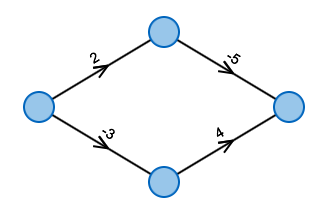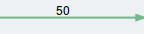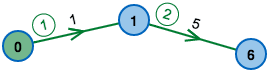What's the cheapest way from left to right?

# Shortest paths and cheapest paths

In many applications one wants to obtain the shortest path from a to b. Depending on the context, the length of the path does not necessarily have to be the length in meter or miles: One can as well look at the cost or duration of a path – therefore looking for the cheapest path.

## LegendNodeEdge with weight 50

## Which graph do you want to execute the algorithm on?

### Modify it to your desire:

• To create a node, make a double-click in the drawing area.
• To create an edge, first click on the output node and then click on the destination node.
• Right-clicking deletes edges and nodes.

A occured when reading from file: the contents:

## LegendStarting node from where distances and shortest paths are computed."Predecessor edge" that is used by the shortest path to the node.

## Algorithm status

BEGIN

d(v) ← 0

FOR j = 2,..,n DO

d(v[j]) ← ∞

FOR i = 1,..,(|V|-1) DO

FOR ALL (u,v) in E DO

d(v) ← min(d(v), d(u)+l(u,v))

FOR ALL (u,v) in E DO

IF d(v) > d(u) + l(u,v) DO

Message: "Negative Circle"

END

### Variable status:

i d(u) d(v) l(u,v)

## Task is terminated if the tab is changed.

You can open another browser window for reading the description in parallel.

## LegendStarting node from where distances and shortest paths are computed."Predecessor edge" that is used by the shortest path to the node.

## Test your knowledge: How does the algorithm decide?

BEGIN

d(v) ← 0

FOR j = 2,..,n DO

d(v[j]) ← ∞

FOR i = 1,..,(|V|-1) DO

FOR ALL (u,v) in E DO

d(v) ← min(d(v), d(u)+l(u,v))

FOR ALL (u,v) in E DO

IF d(v) > d(u) + l(u,v) DO

Message: "Negative Circle"

END

## The task is terminated if the tab is changed.

You can open another browser window for reading the description in parallel.

## LegendStarting node from where distances and shortest paths are computed.Edge that has already been selected.Edge that has been selected in the previous step.

## What is the optimal ordering of the edges?

The Bellman-Ford Algorithm can compute all distances correctly in only one phase.

To do so, he has to look at the edges in the right sequence. This ordering is not easy to find – calculating it takes the same time as the Bellman-Ford Algorithm itself.As one can see in the example: The ordering on the left in reasonable, after one phase the algorithm has correctly determined all distances. This is not the case on the right.

In this exercise you can test how many phases the algorithm needs for different sequences of the edges.

## Task is terminated if the tab is changed.

You can open another browser window for reading the description in parallel.